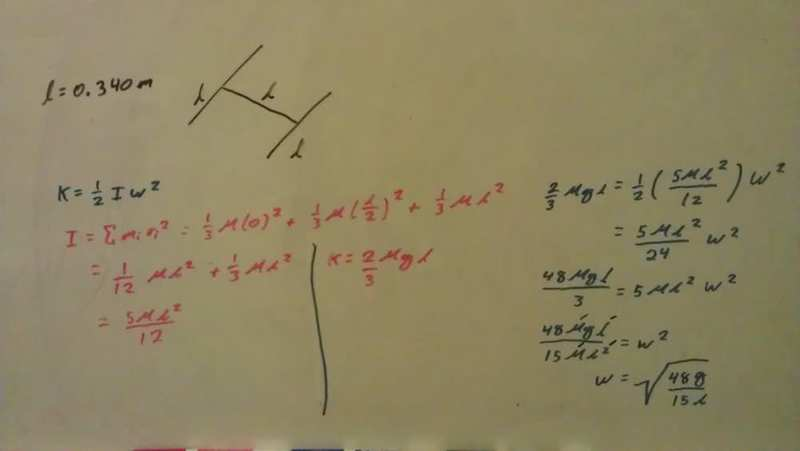# A rigid H falls rotating about one of its legs. What's its angular velocity?

• DavidAp
In summary, the conversation discusses a problem involving a rigid body in the shape of an "H" falling and rotating about one of its legs. The question is about finding the angular velocity of the body when the plane of the "H" is vertical. The conversation includes calculations and discussions about the potential energy and moment of inertia of the body. The correct answer for the angular velocity is 8.05 rad/s.

#### DavidAp

A rigid "H" falls rotating about one of its legs. What's its angular velocity?

A rigid body is made of three identical thin rods, each with length L = 0.340 m, fastened together in the form of a letter H, as suggested by the figure here. The body is free to rotate about a horizontal axis that runs along the length of one of the legs of the H. The body is allowed to fall from rest from a position in which the plane of the H is horizontal. What is the angular speed of the body when the plane of the H is vertical

I knew k = 1/2 Iw^2 so I went on to find k and I about each rod and adding them together to get their total kinetic energy(potential energy)/Inertia. My work is shown below.When I plug this in though I get 9.60 "rad"/s. Why, what did I do wrong? After working on this problem for over an hour I (although my work doesn't show it, it took my a long time to get to this stage) I decided to us I = Icm + Mh^2, knowing that h=l, giving me a new inertia of (17M(L^2))/(12) but when plugging in I got 5.208 "rad"/s.

What am I doing wrong? How do you solve this problem?
Thank you for taking the time to review my question.

Last edited:

The moment of the cross rod is 1/3 mL^2, and that of the leg is 1/12 mL^2+mL^2, according to the Parallel Axis Theorem-17/12 mL^2 altogether.

The potential energy is wrong, it is not 2/3 mLg. Correct.

ehild

ehild said:
The moment of the cross rod is 1/3 mL^2, and that of the leg is 1/12 mL^2+mL^2, according to the Parallel Axis Theorem-17/12 mL^2 altogether.

The potential energy is wrong, it is not 2/3 mLg. Correct.

ehild
Hi there!

I know it's been a while since I've posted this but I've been scratching my head and I can't figure out what PE is. I was thinking that, after taking another look at the problem, the PE for the center rod is mg(l/2) because that's where it's center of mass is, but I'm not sure what the PE for the other rod is. I thought it would just be mgl making the total PE 3/2 mgl (ironically the reciprocal of what I originally thought PE was) but that didn't give me the right answer either.

Any hints on what I'm doing wrong or where I should look for the right answer?

We both fell into the same trap when calculating the moment of inertia of the leg. The axis of rotation is parallel with the rod, so the moment of inertia with respect to the CM of the leg is zero instead of 1/12 mL^2 (that would be true for the axis perpendicular to the rod).

So the total moment of inertia is 1/3 mL^2+mL^2.

ehild